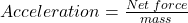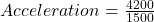## A 1,500-kg truck has a net force of 4,200 N acting on it. What is the truck’s acceleration?

Question

A 1,500-kg truck has a net force of 4,200 N acting on it. What is the truck’s acceleration?

in progress 0
5 months 2021-08-24T21:54:54+00:00 1 Answers 11 views 0

Acceleration = 2.8 m/s²

Explanation:

Given the following data;

Mass = 1500 kg

Net force = 4200 Newton

To find the truck’s acceleration;

Newton’s Second Law of Motion states that the acceleration of a physical object is directly proportional to the net force acting on the physical object and inversely proportional to its mass.

Mathematically, it is given by the formula;Substituting into the formula, we have;Acceleration = 2.8 m/s²